Question

Two identical charged metallic sphares are separated by 7.6 cm has attractive electrostatic force between them. They are made to touch each other and seperated to 7.6 cm distance again. As a result the force turns to repulsive and 10 times smaller in magnitude than the earlier attractive force. If 87 micro C charge is exchanged during this process, find the magnitude of charge (in micro C) left in each sphere at the end.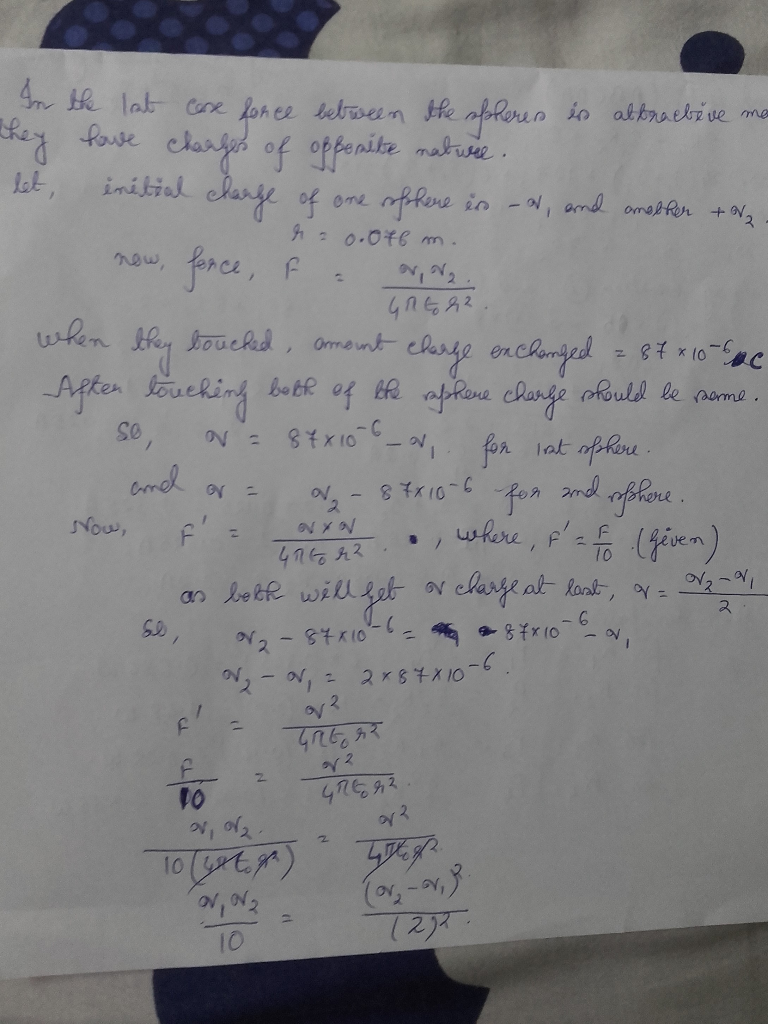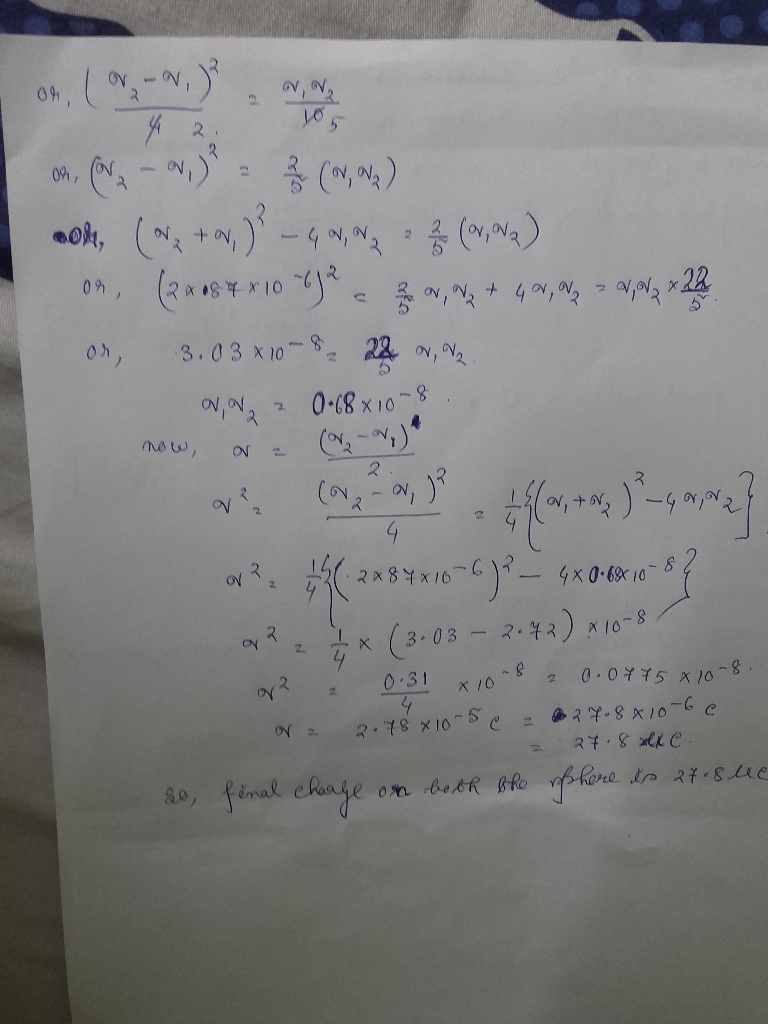#### Earn Coins

Coins can be redeemed for fabulous gifts.

Similar Homework Help Questions
• ### Do each question separately and show step by step Two spherical objects are separated by a...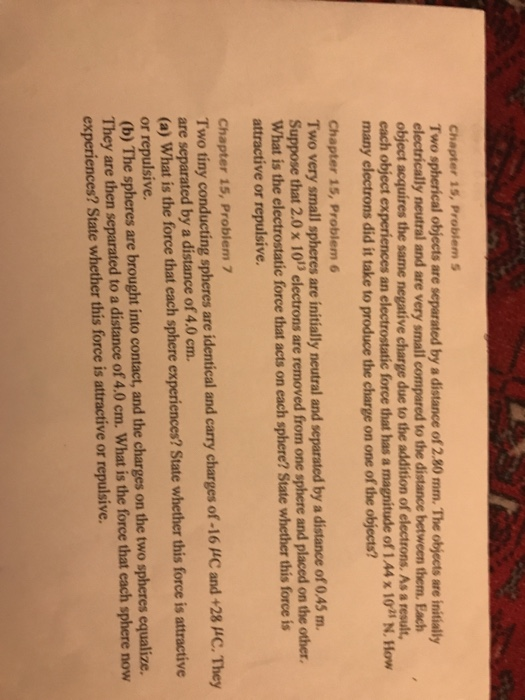Do each question separately and show step by step Two spherical objects are separated by a distance of 2.80 mm. The objects are initially electrically neutral and are very small compared to the distance between them. Each object acquires the same negative charge due to the addition of electrons, As a result, each object experiences an electrostatic force that has a magnitude of 1.44 times 10^-25 N. How many electrons did it take to produce the charge on one of...

• ### The magnitude of the electrostatic force between two identical ions that are separated by a...

The magnitude of the electrostatic force between two identical ions that are separated by a distance of 7.2 × 10-10 m is 75.23 × 10-9 N. (a) What is the charge (inCoulombs) of each ion? (b) How many electrons are “missing” from each ion (thus giving the ion its charge imbalance)?

• ### The electrostatic force between two negatively charged cubes, 10 cm. on each side, with Q1= -7...

The electrostatic force between two negatively charged cubes, 10 cm. on each side, with Q1= -7 µC and Q2= -4 µC is: A) Attractive. The force on cube 1 is twice as large as the force on cube 2. B) Attractive. The force on cube 1 is equal to the force on cube 2. C) Zero. D) Repulsive. The force on cube 1 is twice as large as the force on cube 2. E) Repulsive. The force on cube 1...

• ### The magnitude of the electrostatic force between two identical ions that are separated by 5*10^-10m is...

The magnitude of the electrostatic force between two identical ions that are separated by 5*10^-10m is 3.7*10^-9N. What is the charge on each ion?

• ### The magnitude of the electrostatic force between two identical ions that are separated by a distance...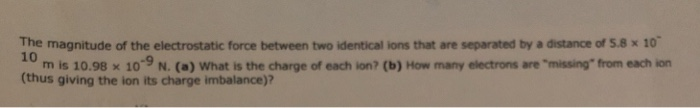The magnitude of the electrostatic force between two identical ions that are separated by a distance of 5.8x 10 0 m is 10.98 x 10-9 N. (a) What is the charge of each ton? (b) How many electrons are "missing" from each ion (thus giving the ion its charge imbalance)?

• ### Two tiny conducting spheres are identical and carry charges of -21.6 µC and +45.5 µC. They...

Two tiny conducting spheres are identical and carry charges of -21.6 µC and +45.5 µC. They are separated by a distance of 2.37 cm. (a) What is the magnitude of the force that each sphere experiences, and is the force attractive or repulsive? (b) The spheres are brought into contact and then separated to a distance of 2.37 cm. Determine the magnitude of the force that each sphere now experiences, and state whether the force is attractive or repulsive.

• ### Two tiny conducting spheres are identical and carry charges of -21.8 µC and +47.5 µC. They...

Two tiny conducting spheres are identical and carry charges of -21.8 µC and +47.5 µC. They are separated by a distance of 2.71 cm. (a) What is the magnitude of the force that each sphere experiences, and is the force attractive or repulsive? N (b) The spheres are brought into contact and then separated to a distance of 2.71 cm. Determine the magnitude of the force that each sphere now experiences, and state whether the force is attractive or repulsive.

• ### Two tiny conducting spheres are identical and carry charges of -21.6 µC and +45.5 µC. They...

Two tiny conducting spheres are identical and carry charges of -21.6 µC and +45.5 µC. They are separated by a distance of 2.37 cm. (a) What is the magnitude of the force that each sphere experiences, and is the force attractive or repulsive? (b) The spheres are brought into contact and then separated to a distance of 2.37 cm. Determine the magnitude of the force that each sphere now experiences, and state whether the force is attractive or repulsive.

• ### Two tiny conducting spheres are identical and carry charges of .2 1.0 μC and +S2.0 pc....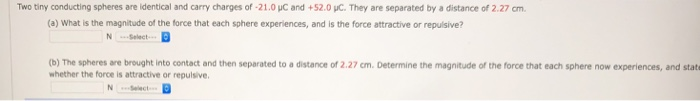Two tiny conducting spheres are identical and carry charges of .2 1.0 μC and +S2.0 pc. They are separated by a distance of 2.27 cm. (a) What is the magnitude of the force that each sphere experiences, and is the force attractive or repulsive? N .Select (b) The spheres are brought into contact and then separated to a distance of 2.27 cm. Determine the magnitude of the force that each sphere now experiences, and stat whether the force is attractive...

• ### The magnitude of the electrostatic force between two identical ions that are separated by a distance...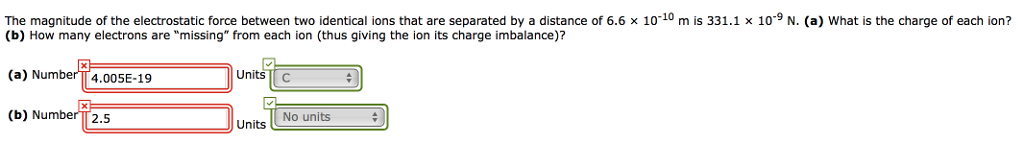The magnitude of the electrostatic force between two identical ions that are separated by a distance of 6.6 x 1010 m is 331.1 x 10-9 N. (a) What is the charge of each ion? (b) How many electrons are "missing" from each ion (thus giving the ion its charge imbalance)? (a) NumberT 4.005E-19 (b) NumberT 2.5 Units C - u Units No units